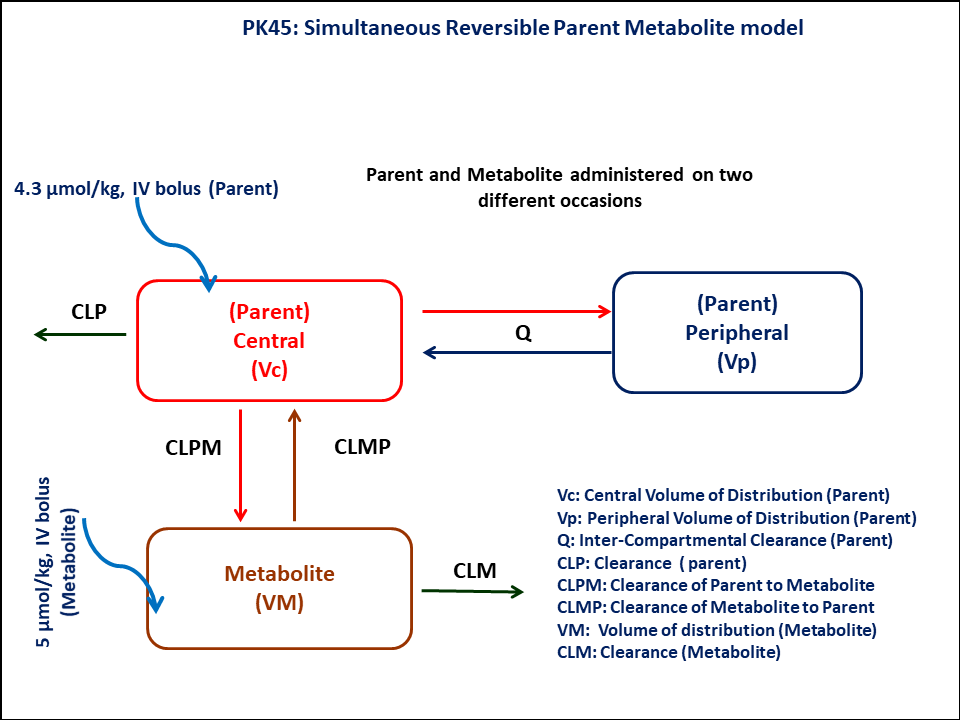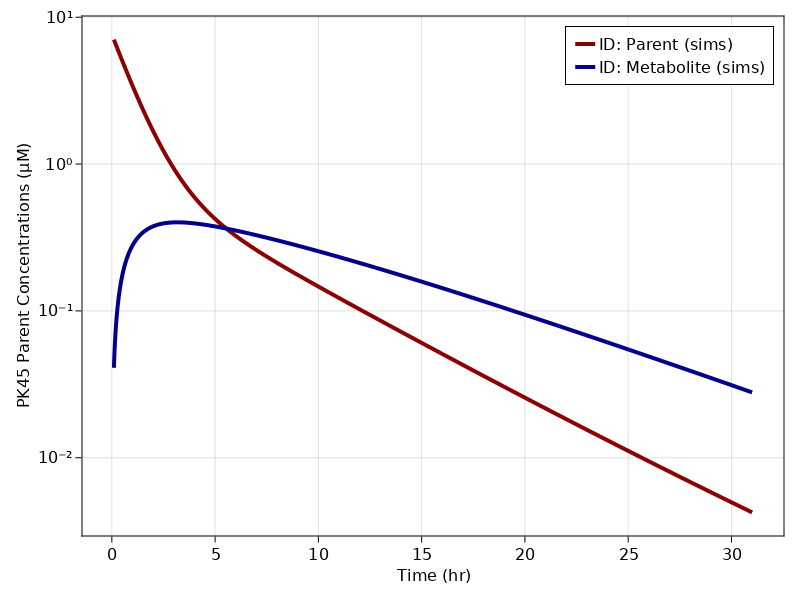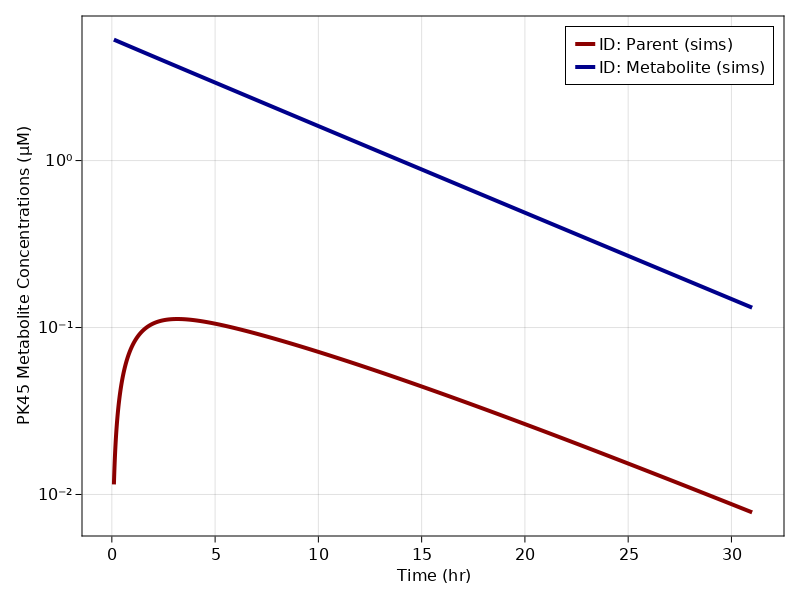# Exercise PK45 - Reversible metabolism of drug A & its metabolite B

### Background

• Structural model - Two compartment model for parent and one compartment Model for metabolite with reversible metabolism

• Route of administration - Administration of parent drug and metabolite on two different occasions

• Dosage Regimen - 4.3 Micromol/Kg of parent & 5 Micromol/Kg of metabolite

• Number of Subjects - 1### Learning Outcome

In this model, you will learn how to build a two compartment parent and one compartment metabolite model with reversible metabolism, while parent and metabolite is administered on two different occasions

### Libraries

Call the "necessary" libraries to get start.

using Random
using Pumas
using PumasUtilities
using CairoMakie


### Model

Two compartment model for parent and one compartment model metabolite

pk_45            = @model begin
desc         = "Two Compartment Model with Metabolite Compartment"
timeu        = u"hr"
end

@param begin
"Volume of Distribution - Central Parent (L/kg)"
tvvcp        ∈ RealDomain(lower=0)
"Volume of Distribution - Peripheral Parent (L/kg)"
tvvpp        ∈ RealDomain(lower=0)
"Intercompartmental Clearance - Parent (L/hr/kg)"
tvqp         ∈ RealDomain(lower=0)
"Clearance - Parent (L/hr/kg)"
tvclp        ∈ RealDomain(lower=0)
"Volume of Distribution - Metabolite Parent (L/kg)"
tvvcm        ∈ RealDomain(lower=0)
"Clearance - Metabolite (L/hr/kg)"
tvclm        ∈ RealDomain(lower=0)
"Conversion of Parent to Metabolite (L/hr/kg)"
tvclpm       ∈ RealDomain(lower=0)
"Conversion of Metabolite to Parent (L/hr/kg)"
tvclmp       ∈ RealDomain(lower=0)
Ω            ∈ PDiagDomain(8)
"Proportional RUV"
σ²_prop      ∈ RealDomain(lower=0)
end

@random begin
η            ~ MvNormal(Ω)
end

@pre begin
Vcp          = tvvcp * exp(η)
Vpp          = tvvpp * exp(η)
Qp           = tvqp * exp(η)
Clp          = tvclp * exp(η)
Vcm          = tvvcm * exp(η)
Clm          = tvclm* exp(η)
Clpm         = tvclpm * exp(η)
Clmp         = tvclmp * exp(η)
end

@dynamics begin
Centralp'    = (Qp/Vpp)*Peripheralp - (Qp/Vcp)*Centralp - (Clp/Vcp)*Centralp - (Clpm/Vcp)*Centralp + (Clmp/Vcm)*Centralm
Peripheralp' = (Qp/Vcp)*Centralp -  (Qp/Vpp)*Peripheralp
Centralm'    = -(Clm/Vcm)*Centralm - (Clmp/Vcm)*Centralm + (Clpm/Vcp)*Centralp
end

@derived begin
cp           = @. Centralp/Vcp
met          = @. Centralm/Vcm
"""
Observed Concentration - Parent (uM)
"""
dv_cp        ~ @. Normal(cp, sqrt(cp^2*σ²_prop))
"""
Observed Concentration - Metabolite (uM)
"""
dv_met       ~ @. Normal(met, sqrt(cp^2*σ²_prop))
end
end

PumasModel
Parameters: tvvcp, tvvpp, tvqp, tvclp, tvvcm, tvclm, tvclpm, tvclmp, Ω, σ
²_prop
Random effects: η
Covariates:
Dynamical variables: Centralp, Peripheralp, Centralm
Derived: cp, met, dv_cp, dv_met
Observed: cp, met, dv_cp, dv_met


### Parameters

Parameters provided for simulation. tv represents the typical value for parameters.

• $tvvcp$ - Volume of distribution of central compartment of Parent (L/kg)

• $tvvpp$ - Volume of distribution of peripheral compartment of Parent (L/kg)

• $tvqp$ - Intercompartmental clearance of Parent (L/hr/kg)

• $tvclp$ - Clearance of Parent (L/hr/kg)

• $tvvcm$ - Volume of distribution of central compartment of Metabolite (L/kg)

• $tvclm$ - Clearance of Metabolite (L/hr/kg)

• $tvclpm$ - Conversion of Parent to Metabolite (L/hr/kg)

• $tvclmp$ - Conversion of Metabolite to Parent (L/hr/kg)

• $Ω$ - Between Subject Variability,

• $σ$ - Residual error

param = (tvvcp   = 0.563,
tvvpp   = 0.424,
tvqp    = 0.115,
tvclp   = 0.343,
tvvcm   = 0.932,
tvclm   = 0.068,
tvclpm  = 0.015,
tvclmp  = 0.046,
Ω       = Diagonal([0.0,0.0,0.0,0.0,0.0,0.0,0.0,0.0]),
σ²_prop = 0.001)

(tvvcp = 0.563, tvvpp = 0.424, tvqp = 0.115, tvclp = 0.343, tvvcm = 0.932,
tvclm = 0.068, tvclpm = 0.015, tvclmp = 0.046, Ω = [0.0 0.0 … 0.0 0.0; 0.0
0.0 … 0.0 0.0; … ; 0.0 0.0 … 0.0 0.0; 0.0 0.0 … 0.0 0.0], σ²_prop = 0.001)


### Dosage Regimen

• A dose of 4.3 μmol/kg of the Parent is administered as a rapid IV Injection over 15 seconds

• A dose of 5 μmol/kg of the metabolite is administered as a rapid IV Injection over 15 seconds

dr_p  = DosageRegimen(4.3,cmt = 1,time = 0, duration = 0.0041)
sub_p = Subject(id = "Parent", events = dr_p)
dr_m  = DosageRegimen(5, cmt = 3, time = 0, duration = 0.0041)
sub_m = Subject(id = "Metabolite", events = dr_m)
sub   = [sub_p, sub_m]

Population
Subjects: 2
Observations:


### Simulation

We are going to simulate parent and metabolite concentration profile

Random.seed!(123)
sim_sub = simobs(pk_45, sub, param, obstimes = 0.1:0.001:31)


### Visualization

f1, a1, p1 = sim_plot(pk_45, sim_sub,
observations = :cp,
color = :redsblues,
linewidth = 4,
axis = (xlabel = "Time (hr)",
ylabel = "PK45 Parent Concentrations (μM)",
xticks = 0:5:35, yscale = log10))
axislegend(a1)
f1f2, a2, p2 = sim_plot(pk_45, sim_sub,
observations = :met,
color = :redsblues,
linewidth = 4,
axis = (xlabel = "Time (hr)",
ylabel = "PK45 Metabolite Concentrations (μM)",
xticks = 0:5:35, yscale = log10))
axislegend(a2)
f2